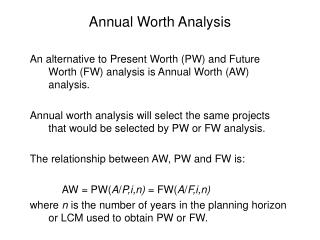DownloadDownload PresentationAnnual Worth Analysis

Annual Worth Analysis

Download PresentationAnnual Worth Analysis

- - - - - - - - - - - - - - - - - - - - - - - - - - - E N D - - - - - - - - - - - - - - - - - - - - - - - - - - -
Presentation Transcript

1. Annual Worth Analysis An alternative to Present Worth (PW) and Future Worth (FW) analysis is Annual Worth (AW) analysis. Annual worth analysis will select the same projects that would be selected by PW or FW analysis. The relationship between AW, PW and FW is: AW = PW(A/P,i,n) = FW(A/F,i,n) where n is the number of years in the planning horizon or LCM used to obtain PW or FW.

2. Annual Worth Analysis Annual Worth (AW) analysis is often desirable since an annual worth is often more intuitive to individuals who think in terms of annual cash flows.

3. Annual Worth Analysis Annual Worth (AW) analysis is also desirable since AW has to be calculated for only one life cycle. It is not necessary to use LCM as we did for the PW and FW analyses. When alternative have different lives, the AW method make the following assumptions: 1. The services provided are needed for the indefinite future (forever) 2. The selected alternative will be repeated for succeeding life cycles in exactly the same manner as for the first life cycle. 3. All cash flows will have the same estimated values in every life cycle.

4. Annual Worth Analysis Earlier PW analysis for different-life alternatives: Service Example: You are evaluating the purchase of a new or used cars that needs to last you for 10 years. i = 8%. New CarUsed Car Purchase Price \$20,000 \$10,000 Annual Maint. \$ 750 \$ 1,000 Resale Value \$ 4,000 \$ 4,000 Life, years 10 5 PW (LCM 10) -\$23,180 -\$18,941 AW(New) = -\$20,000(A/P,8%,10) - \$750 + \$4000(A/F,8%,10) = -\$3454.48 AW(Used) = -\$10,000(A/P,8%,5) - \$1000 + \$4000(A/F,8%,5) = -\$2822.76 What is AW is calculate using the PW (LCM 10) values?

5. Capital Recovery Capital Recovery (CR) is the equivalent annual cost of owning an asset plus the return on the initial investment. Consider an alternative where P is the total of all initial investments, A is the equivalent of all annual cash flows (cost only in a service project, receipts and costs in revenue projects), and S is the salvage value. Then the relationship between CR and AW is: AW = CR + A So that CR = -[P(A/P,i,n) - S(A/F,i,n)].

6. Capital Recovery Example: You are evaluating the purchase of a two income properties. You expect a MARR of 15%. What is the Annual Worth? \$75K Home Purchase Price \$15,000 Annual Maint. \$ 6,000 Annual Income \$ 7,500 Resale (after Expenses) \$90,000 Life, years 15 CR = -\$15,000(A/P,15%,15) + \$90,000(A/F,15%,15) = -\$673.50 (in otherwords, you must make \$673.50 per year to make your MARR or to recover your capital at 15% interest) A = \$1,500 AW = CR + A = \$826.50

7. Evaluation Alternative Using AW To evaluate alternatives: First, calculate the AW at the MARR. For – One alternative: If AW > 0, then the MARR is met or exceeded. Two or more alternatives: Choose the project with lowest cost AW value (service projects) of highest income (revenue projects) AW value.

8. Evaluation Alternative Using AW Service Example: You are evaluating the purchase of a new or used cars that needs to last you for 10 years. MARR = 8%. New CarUsed Car Purchase Price \$20,000 \$10,000 Annual Maint. \$ 750 \$ 1,000 Resale Value \$ 4,000 \$ 4,000 Life, years 10 5 AW(new) = -\$20,000(A/P,8%,10) -\$750 + \$4000(A/F,8%,10) AW(new) = -\$3454.48 AW(used) = -\$10,000(A/P,8%,5) - \$1000 + \$4000(A/F,8%,5) AW(used) = -\$2822.76

9. AW of A Permanent Investment For a permanent investment with an initial investment of P, the capital recovery is simply the annual interest earned, in other words: CR = Pi In addition, to find the annual worth, all cash flows must be converted to equivalent annual amounts, A. Then, AW = CR + A

10. AW of A Permanent Investment Example: A toll-road was just completed at a cost of \$1.5 billion, with major maintenance expenditures of \$500 million forecast every 10 years. Annual receipts minus maintenance results in a positive cash flow of \$150 million. What is the annual worth, assuming i = 5%? \$150 \$150 \$150 0 1 2 3 4 5 6 7 8 9 10 11 … 20 \$500 \$500 \$1500 CR = \$1500 * .05 = \$75 million AW = -\$75 + 150 - \$500(A/F,5%,10) = \$35.25 million